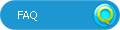# HotmathTitle:
Hotmath
Author:
Hotmath
Chapter: Real Numbers and the Pythagorean Theorem Section: The Pythagorean Theorem and its Converse

Problem: 1

Identify the legs and the hypotenuse of the given right triangle.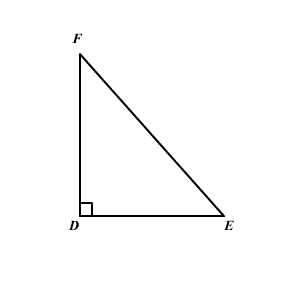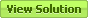Problem: 3

A leg of an isosceles triangle is 21 in. long. What is the length of the hypotenuse? Round the answer to the nearest tenth.Problem: 5

A leg of an isosceles triangle is 11.25 long. What is the length of the hypotenuse? Round the answer to the nearest tenth.Problem: 7

Let a and b are the measures of the legs and c is the measure of the hypotenuse in a right triangle. Find the missing measure. If necessary, round decimal answers to the nearest tenth.

a = 33, b = 44Problem: 9

Let a and b are the measures of the legs and c is the measure of the hypotenuse in a right triangle. Find the missing measure. If necessary, round decimal answers to the nearest tenth.

a = √ 15, c = 8Problem: 11

Obtain the missing length to the nearest tenth of a unit.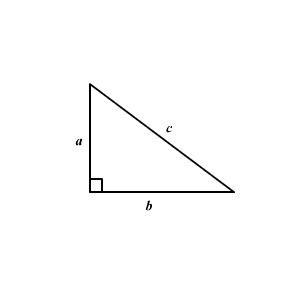Problem: 13

What is the length of the hypotenuse?Problem: 15

Form an equation for x and then solve for x . Then round decimal answers to the nearest tenth.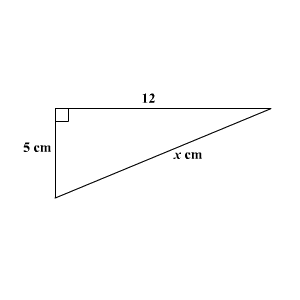Problem: 17

Obtain the missing length to the nearest tenth of a unit.Problem: 19

Obtain the missing length to the nearest tenth of a unit.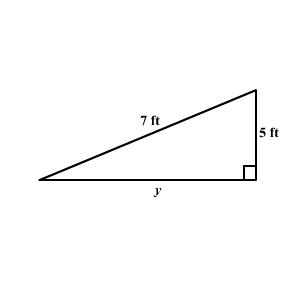Problem: 21

Obtain the value of n in the given diagram. Give the answer as a square root.Problem: 23

Draw a right triangle with the given length of the sides if possible.

7, 24, 25Problem: 25

Determine the Pythagorean Triples using the values, m = 7 and n = 4.Problem: 27

Any three positive integers that make a 2 + b 2 = c 2 form a Pythagorean triple. If each number in the set is multiplied by 3, will the new numbers form a Pythagorean triple? Verify the answer.

6, 8, 10Problem: 29

The sides of a triangle measure 8, 15, and 17. Is the triangle a right triangle?Problem: 31

Check whether the triangle is a right triangle. The dimensions are:

6 ft, 7 ft, 13 ftProblem: 33

Identify whether a right triangle can be formed with the three lengths given. Show your work.

2 m, 0.94 m, 0.96 mProblem: 35

Using the converse of the Pythagorean theorem, state whether the triangle is a right triangle.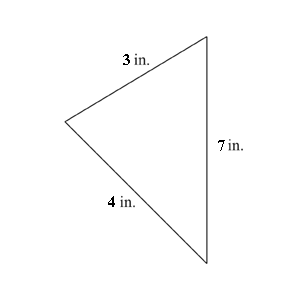Problem: 37

The sides of a triangle measure a , b , and c . Use the table to find the type of the triangle.Problem: 39

Determine the perimeter and area of the given figure.Problem: 41

The opposite sides of a quadrilateral are congruent. The sides measure 5 in. and 7 in. and the diagonal measures √ 74. Is the quadrilateral a rectangle?Problem: 43

David travels 15 kilometers north, 16 kilometers west, and then 15 kilometers north again. Find the distance traveled by him from the starting point.